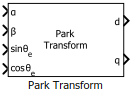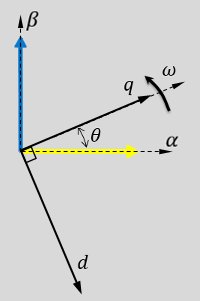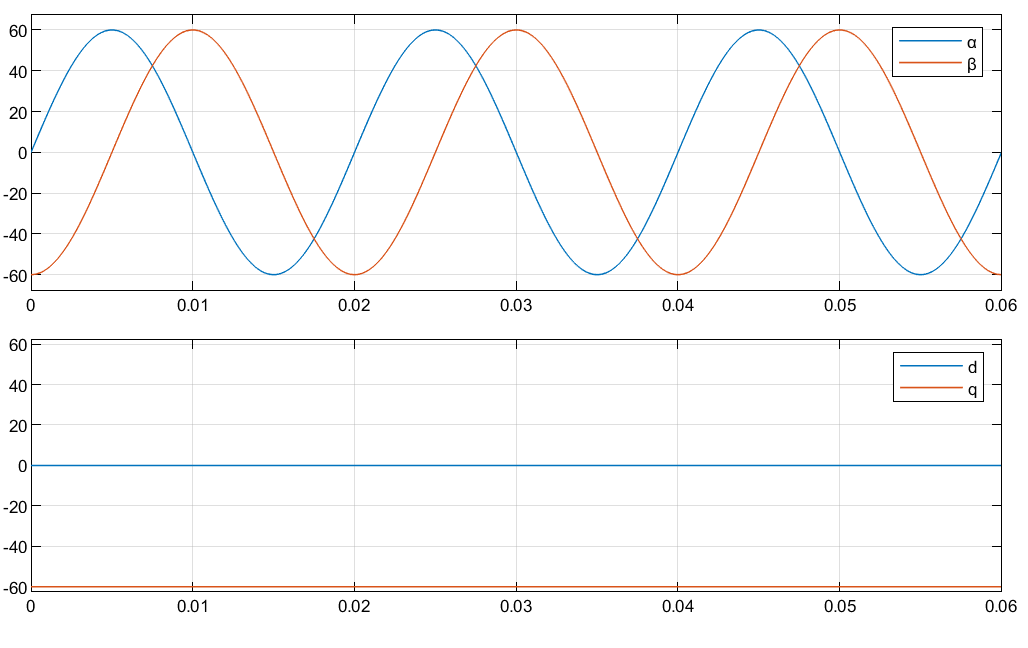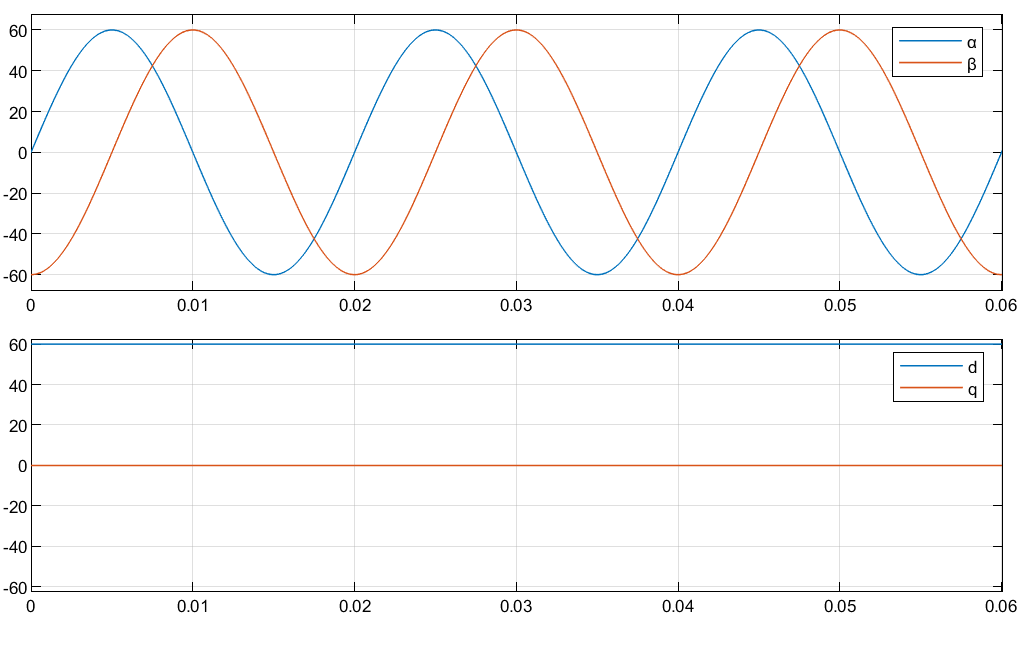# Park Transform

Implement αβ to dq transformation

Since R2020a

•Libraries:
Motor Control Blockset / Controls / Math Transforms
Motor Control Blockset HDL Support / Controls / Math Transforms

## Description

The Park Transform block computes the Park transformation of two-phase orthogonal components (α, β) or multiplexed αβ0 components in a stationary αβ reference frame.

The block accepts the following inputs:

• Either α-β axes components or multiplexed components αβ0 in the stationary reference frame. Use the Number of inputs parameter to use either two or three inputs.

• Sine and cosine values of the corresponding angles of transformation.

When using two-input configuration, it outputs orthogonal direct (d) and quadrature (q) axis components in the rotating dq reference frame. When using three-input configuration, it outputs multiplexed components dq0.

For a balanced system, the zero component is equal to zero.

You can configure the block to align either the d- or the q-axis with the α-axis at time t = 0.

The figures show the α-β axes components in an αβ reference frame and a rotating dq reference frame for when:

• The d-axis aligns with the α-axis.• The q-axis aligns with the α-axis.In both cases, the angle θ = ωt, where:

• θ is the angle between the α- and d-axes for the d-axis alignment or the angle between the α- and q-axes for the q-axis alignment. It indicates the angular position of the rotating dq reference frame with respect to the α-axis.

• ω is the rotational speed of the d-q reference frame.

• t is the time, in seconds, from the initial alignment.

The figures show the time-response of the individual components of the αβ and dq reference frames when:

• The d-axis aligns with the α-axis.• The q-axis aligns with the α-axis.### Equations

The following equations describe how the block implements Park transformation.

• When the d-axis aligns with the α-axis.

• When the q-axis aligns with the α-axis.

where:

• ${f}_{\alpha }$ and ${f}_{\beta }$ are the two-phase orthogonal components in the stationary αβ reference frame.

• ${f}_{d}$ and ${f}_{q}$ are the direct and quadrature axis orthogonal components in the rotating dq reference frame.

## Ports

### Input

expand all

Alpha-axis component, α, in the stationary αβ reference frame.

#### Dependencies

To enable this port, set the Number of inputs parameter to `Two inputs`.

Data Types: `single` | `double` | `fixed point`

Beta-axis component, β, in the stationary αβ reference frame.

#### Dependencies

To enable this port, set the Number of inputs parameter to `Two inputs`.

Data Types: `single` | `double` | `fixed point`

Multiplexed alpha-axis component, α and beta-axis component, β and `0` component, in the stationary αβ reference frame.

#### Dependencies

To enable this port, set the Number of inputs parameter to `Three inputs`.

Data Types: `single` | `double` | `fixed point`

Sine value of the angle of transformation, θe. θe is the angle between the rotating reference frame and the α-axis.

Data Types: `single` | `double` | `fixed point`

Cosine value of the angle of transformation, θe. θe is the angle between the rotating reference frame and the α-axis.

Data Types: `single` | `double` | `fixed point`

### Output

expand all

Direct axis component, d, in the rotating dq reference frame.

#### Dependencies

To enable this port, set the Number of inputs parameter to `Two inputs`.

Data Types: `single` | `double` | `fixed point`

Quadrature axis component, q, in the rotating dq reference frame.

#### Dependencies

To enable this port, set the Number of inputs parameter to `Two inputs`.

Data Types: `single` | `double` | `fixed point`

Multiplexed direct axis component, d, quadrature axis component, q, and the 0 component in the rotating dq reference frame.

#### Dependencies

To enable this port, set the Number of inputs parameter to `Three inputs`.

Data Types: `single` | `double` | `fixed point`

## Parameters

expand all

Select the number of inputs that you can specify:

• `Two inputs` — Configure the block to accept two separate input signals α and β. The block generates two separate output signals d and q.

• `Three inputs` — Configure the block to accept a multiplexed input containing α, β, and 0 signals. The block generates a multiplexed output containing d,q, and 0 signals.

Align either the d- or q-axis of the rotating reference frame to the α-axis of the stationary reference frame.

Type of position (theta) input:

• `Sine and Cosine electrical position` — Configure the block to directly accept sinθe and cosθe inputs.

• `Electrical position` — Configure the block to accept the electrical position (θe) input. The block internally computes the sinθe and cosθe signals from the θe input.

Unit of the electrical position input (θe).

#### Dependencies

To enable this parameter, set Theta input to `Electrical position`.

Size of the lookup table array that the block uses to compute sinθe and cosθe signals from the θe input. You can specify a value between 125 and 4095.

#### Dependencies

To enable this parameter, set Theta input to `Electrical position`.

## Version History

Introduced in R2020a